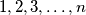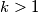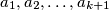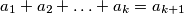### MEMO 2007 pojedinačno problem 2

Kvaliteta:
Avg: 3,3
Težina:
Avg: 6,0
A set of balls contains$n$ balls which are labeled with numbers$1,2,3,\ldots,n$. We are given$k > 1$ such sets. We want to colour the balls with two colours, black and white in such a way, that

(a) the balls labeled with the same number are of the same colour,

(b) any subset of$k+1$ balls with (not necessarily different) labels$a_{1},a_{2},\ldots,a_{k+1}$ satisfying the condition$a_{1}+a_{2}+\ldots+a_{k}= a_{k+1}$, contains at least one ball of each colour.

Find, depending on$k$ the greatest possible number$n$ which admits such a colouring.
Izvor: Srednjoeuropska matematička olimpijada 2007, pojedinačno natjecanje, problem 2